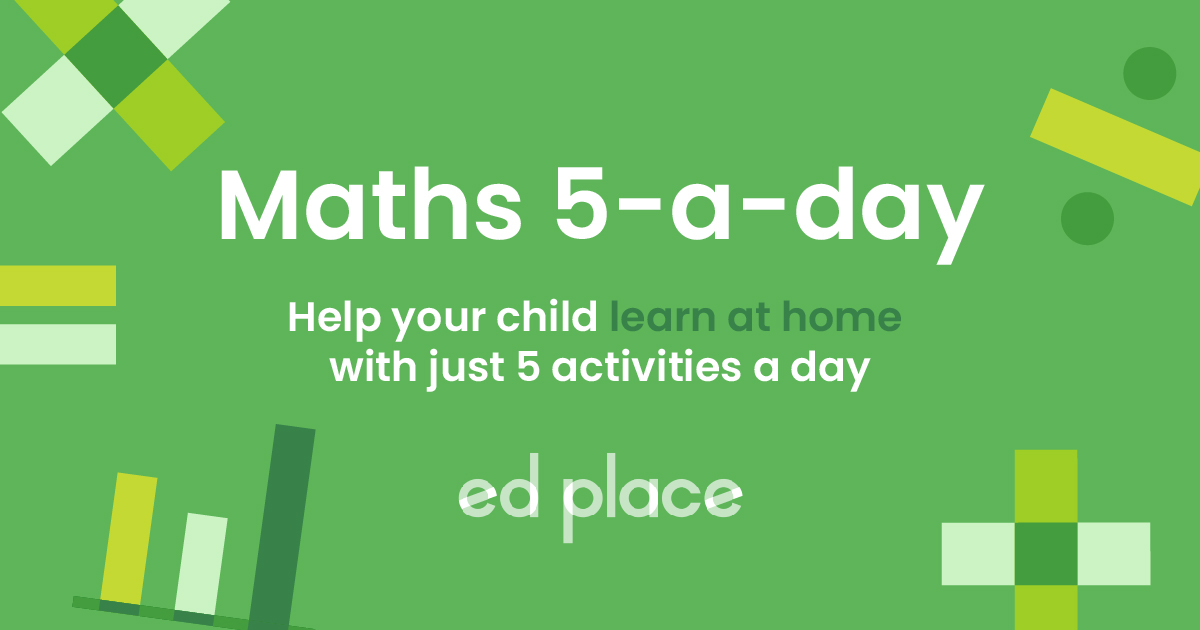# EdPlace's Year 4 Home Learning Maths Lesson: Area

Looking for short lessons to keep your child engaged and learning? Our experienced team of teachers have created English, maths and science lessons for the home, so your child can learn no matter where they are.  And, as all activities are self-marked, you really can encourage your child to be an independent learner. Get them started on the lesson below and then jump into our teacher-created activities to practice what they've learnt. We've recommended five to ensure they feel secure in their knowledge - 5-a-day helps keeps the learning loss at bay (or so we think!).

Are they keen to start practising straight away? Head to the bottom of the page to find the activities.

Now...onto the lesson!

Key Stage 2 Statutory Requirements for Maths
Year 4 students should be able to find the area of rectilinear shapes by counting squares.

# Can your child confidently find the area of different shapes?

Area is a key concept for children to learn by the end of Key Stage 2. It is often taught alongside perimeter and it is very easy to get the two concepts muddled!

At EdPlace we’re surrounded by a team of experts who communicate these concepts with children on a day-to-day basis, and we’re ready to share their teaching gems with you.  Follow the step-by-step approach below to make working with area as easy as pie! Every good lesson has a purpose or an objective. We’re confident by the end of this that your child will be able to:

1) Understand what area is

2) Apply this understanding to find areas of shapes drawn on squared paper

3) Explain how to use their mental maths facts to speed up the process and find areas of more complex shapes quickly and accurately.

## Step 1 - Key terminology

Before we start finding areas of shapes it’s important to check that your child understands what the key terminology means.

The area of a 2D shape is defined by the amount of surface which is covered by the shape. When finding the area of a shape drawn on squared paper, this is very simple. You just count the squares covered by the shape!

## Step 2 - Understand area

In year 3, your child will have been taught to measure the perimeter of shapes. This is the distance around the edge of a shape and children are often taught to count the number of square edges a shape has to find its perimeter.

For example, this shape shown on 1 cm squared paper has a perimeter of 10 cm. This is because the outside of the shape is 10 square edges long.In year 4, the concept of area is introduced and this can sometimes cause confusion for children when they muddle the concepts of area and perimeter since both are commonly explained using shapes drawn on squared paper. In the next section, we will explore how to ensure this concept becomes easy peasy!

## Step 3 - Calculating area

In year 4, children will be shown shapes drawn on squared backgrounds and they will be told what each square represents. This is commonly 1cm² or 1m² but it is good practice to read the question fully to check this.Area is sometimes just counted as squares but is also often represented using a small number 2 just after the measure of unit. This shows that the unit is a squared unit. E.g. The diagram below shows a square with sides all 1cm. The area of this square would be 1cm² or one centimetre squared.If we look again at the shape from step 2, we can see that the area of the shape would be 6cm². This is because the shape covers 6 of the 1cm² squares.This is all very nice and easy when we are calculating or counting the area of squares and rectangles. However, in year 4, your child will begin to work with slightly more complicated shapes. This could include shapes which are a more complex shape like this one:

1 square = 1cm²These can be a little trickier and it can help to divide the shape into more than one piece to ensure the squares are counted accurately.

4 + 12 = 16cm²By splitting the shape up and then adding the two totals together we can find the area.

Another type of shape your child might encounter is like this, with half squares:

1 square = 1cm²4 halves – 2 wholes

2 + 12 = 14cm²We can add the half squares together to make whole ones, as shown above.

## Step 4 - Putting it into practise...

Why not apply the above to the following area questions together?

a) Find the area of the following shape drawn on 1cm squared paper.b) Find the area of the following shape drawn on paper where 1 square represents 1m².c) What is the area of the following shape in cm²?d) Order these shapes from smallest to largest area. Each square is 1cm².## Step 5 - Give it a go...

Now that you’ve covered this lesson together, why not put this to the test and assign your child the following area activities in this order? All activities are created by teachers and automatically marked. Plus, with an EdPlace subscription, we can automatically progress your child at a level that's right for them. Sending you progress reports along the way so you can track and measure progress, together - brilliant!

a) The square has an area of 16cm².

b) The shape has an area of 12m².

c) The shape has an area of 10cm².

d) The correct order is C (4½cm²), A (8cm²), B (9cm²) then D (10cm²).

Keep going! Looking for more activities, different subjects or year groups?

Click the button below to view the EdPlace English, maths, science and 11+ activity library